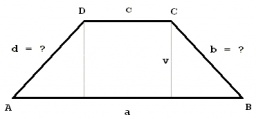# Centimeters 6604

A four-sided prism has a volume of 648 cubic centimeters. The trapezoid that is its base has the dimensions a is equal to 10 centimeters, c is equal to eight centimeters, and height v is equal to 6 centimeters. Calculate the height of the prism.

h =  12 cm

### Step-by-step explanation:Did you find an error or inaccuracy? Feel free to write us. Thank you!

Tips for related online calculators